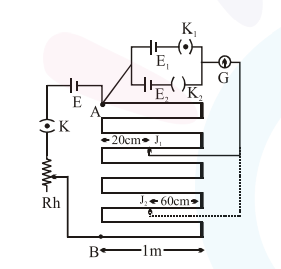# In the given circuit of potentiometer,`
Question:

In the given circuit of potentiometer, the potential difference $\mathrm{E}$ across $\mathrm{AB}$ (10m length) is larger than $\mathrm{E}_{1}$ and $\mathrm{E}_{2}$ as well. For key $\mathrm{K}_{1}$ (closed), the jockey is adjusted to touch the wire at point $\mathbf{J}_{1}$ so that there is no deflection in the

galvanometer. Now the first battery $\left(\mathrm{E}_{1}\right)$ is replaced by second battery $\left(E_{2}\right)$ for working by making $\mathrm{K}_{1}$ open and $\mathrm{K}_{2}$ closed. The galvanometer gives then null deflection at $\mathrm{J}_{2}$.

The value of $\frac{E_{1}}{E_{2}}$ is $\frac{a}{b}$, where $a=$________.Solution:

Length of $\mathrm{AB}=10 \mathrm{~m}$

For battery $\mathrm{E}_{1}$, balancing length is $l_{1}$

$l_{1}=380 \mathrm{~cm} \quad$ [from end $\left.\mathrm{A}\right]$

For battery $\mathrm{E}_{2}$, balancing length is $l_{2}$

$l_{2}=760 \mathrm{~cm} \quad[$ from end $\mathrm{A}]$

Now, we know that $\frac{\mathrm{E}_{1}}{\mathrm{E}_{2}}=\frac{l_{1}}{l_{2}}$

$\Rightarrow \frac{E_{1}}{E_{2}}=\frac{380}{760}=\frac{1}{2}=\frac{a}{b}$

$\therefore a=1 \& b=2 .$

$a=1$